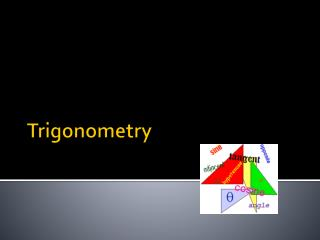DownloadDownload PresentationTrigonometry

Trigonometry

Télécharger la présentationTrigonometry

- - - - - - - - - - - - - - - - - - - - - - - - - - - E N D - - - - - - - - - - - - - - - - - - - - - - - - - - -
Presentation Transcript

1. Trigonometry

2. Goals: Trigonometry Unit • What is Trigonometry? • Origins • 3 Basic Trig Functions: Sine, Cosine, Tangent • Apply Trig Functions to Solve Right Triangle Problems • Applications and Uses

3. What is Trigonometry?

4. What is Trigonometry? TRIANGLE MEASURE GREEK WORD MEANING:

5. Origins

6. Origins of Trigonometry • 1500 BC Egyptians used shadow clocks

7. Greek Origins • The Ancient Greeks further evolved Sundials by erecting a gnomon ( staff) in the ground • Hipparchus used Trigonometry to measure distance to the stars

10. Sine - Cosine - Tangent Trigonometric Ratios

11. Right Triangles RIGHT TRIANGLES MUST HAVE A 90˚ ANGLE LEG OPPOSITE ANGLE Ѳ HYPOTENUSE 90˚ Ѳ LEG ADJACENT TO ANGLE Ѳ

12. Similar Triangle Theorem • If two angles of one triangle are congruent to two angles of another, the triangles are Similar. • Right triangles will be similar if an acute angle of one is equal to an acute angle of the other.

13. Sine of Ѳ length of side OPPOSITE Ѳ length of Hypotenuse LEG OPPOSITE ANGLE Ѳ HYPOTENUSE Ѳ

14. Cosine of Ѳ length of side ADJACENT Ѳ length of Hypotenuse HYPOTENUSE 90˚ Ѳ LEG ADJACENT TO ANGLE Ѳ

15. Tangent of Ѳ length of side OPPOSITE Ѳ length of side ADJACENT Ѳ LEG OPPOSITE ANGLE Ѳ Ѳ LEG ADJACENT TO ANGLE Ѳ

16. Trigonometric Functions length of side OPPOSITE Ѳ length of Hypotenuse Sin of Ѳ= Ѳ length of side ADJACENT Ѳ length of Hypotenuse Cos of Ѳ = length of side OPPOSITE Ѳ length of side ADJACENT Ѳ Tan of Ѳ =

17. SOH-CAH-TOA?

19. Sample Problems 1.) 5 A 4 B Ѳ Ѳ 3 C Find the values of: A.) sin Ø = _______ B.) cos Ø = _______ C.) tan Ø = _______ A.) sin Ø = _____ B.) cos Ø = _____ C.) tan Ø = ____

20. Practice Problems Worksheet

21. Applications - Flag Suppose a Flagpole casts a 12 ft shadow when the sun is at an angle of 64˚ with the ground. What is the height of the pole?

22. Application Problems Group Activity Airplanes – Rockets- Ships Draw a picture Identify What you Know Determine what you Need to know Set up the Ratio Solve for the Answer(s)

23. Airplane A plane takes off from a runway at point A and climbs while maintaining a constant angle with the ground. When plane has traveled 1000 meters, its altitude is 290 meters. 1.Find the angle x, at which the plane has climbed with respect to the ground. 2. How Far from the runway point A would an observer on the ground be?

24. Airplane y 1.Sin x = 290 1000 2. cos x˚ = y 1000

25. Rocket An observer 5.2 km from a launch pad observes a rocket ascending. A. At a particular time the angle of elevation is 37 degrees. How high is the rocket? B. How far is the observer from the rocket? C. What will the angle of elevation be when the rocket reaches 30 km?

26. Rocket B A 37 5.2 A. Tan 37 = A 5.2 B. Cos 37 = 5.2 B C. Tan = 30 Ø 5.2

27. Ship A ship sails 13 miles on a bearing of 30 degrees. A. How far north of its original position is the ship? B. How far east of its original position is the ship? A bearing is the angle measured clockwise from due North.

28. Ship B A A. Cos 30 = 13 A 13 B. Sin 30 = B 13 30˚

29. Homework